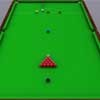#### You may also like### Knock-out

Before a knockout tournament with 2^n players I pick two players. What is the probability that they have to play against each other at some point in the tournament?### Squash

If the score is 8-8 do I have more chance of winning if the winner is the first to reach 9 points or the first to reach 10 points?### Snooker

A player has probability 0.4 of winning a single game. What is his probability of winning a 'best of 15 games' tournament?

# Snooker Frames

### Why do this problem?

This problem gives practice in a relatively complicated probability calculation drawn from a simple situation. It will require clear visualisation of the possibilities, accurate working and a good understanding of permutations and combinations. It gives a good chance to practise calculator skills and the use of the $^nC^r$ button. The context will allow a discussion concerning the role of intuition versus calculation in probability.

### Possible approach

Before beginning this problem ask students whether they think that a weaker player is more or less likely to win over a long match. Can they explain why they believe that this is the case? Are these arguments completely convincing to the rest of the class. Can they estimate how likely they believe that the weaker player is to win over $11$ frames? Perhaps each student could make a guess. The closest guess without going above wins.

In probability, calculation is used to settle disputes. Before students solve the problem they will need to be very clear as to the combinations of matches which lead to a win for the weaker player. They will then need to write this down in a clear way before working out the numbers. Spreadsheets or calculators will be necessary for this. As the calculation is relatively long it will require good calculator or recording skills successfully to obtain the answer, even if the route to the answer is conceptually clear to students. It might provide an opportunity explicitly to practise the use of calculator keys such as ANS, $^nC^r$ and $!$. Can students encode the entire calculation in a single line of calculation?

Once the answer is found, compare with the initial estimates that the class made. Whose intuition was reliable?

### Key questions

Before attempting the computation, can you estimate the chance of the weaker player winning the match?

If the weaker player only had a 4% chance of winning, what do you feel would happen to his chances of winning the match as the number of frames increases?

Which player must win the final frame of the match?

What are the possible final scorelines?

### Possible extension

Can students write down a generalisation of the formula used to show the chance of a player winning a match played over $2n+1$ frames? The numerical investigation will allow students to put their formula into practice.

### Possible support

Reduce the complexity of the calculation: what is the chance of winning a best of $3$ or a best of $5$ match?